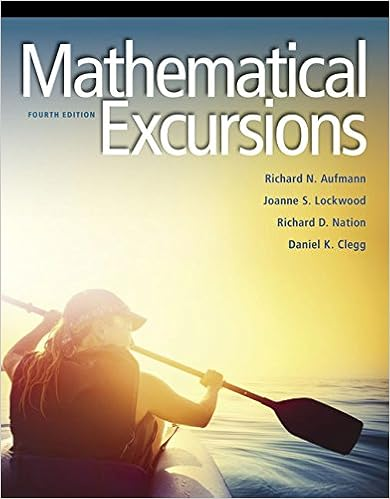# 30 a bin contains 10 red chips 8 white chips and 2

• Notes
• 5

This preview shows page 4 - 5 out of 5 pages.

##### We have textbook solutions for you!
The document you are viewing contains questions related to this textbook.The document you are viewing contains questions related to this textbook.
Chapter 12 / Exercise 3
Mathematical Excursions
AufmannExpert Verified
30. A bin contains 10 red chips, 8 white chips and 2 blue chips. Three chips are selected without replacement. Determine: a) The probability that all three chips are white. b) The probability that all three chips are red. c) The probability that all three chips are blue. 31. Four cards are selected from a deck of cards. Determine the probability that all four of them are hearts. 32. Four cards are selected from a deck of cards. Determine the probability that all four of them are red. 33. Four cards are selected from a deck of cards. Determine the probability that all four of them are Jacks. Section 2.7 34. Use the Venn diagram to answer the probability questions. a) ( | ) P A B b) ( | ) P B A c) ( | ) P A C d) ( | ) P C A e) [ |( )] P A B C f) [( )| ] P B C A g) [ |( )] P A B C h) [( )| ] P B C A i) ( | ) C P B A j) ( | ) C P A B j) [( )|( )] P A B A C k) [( )|( )] P A B A B
##### We have textbook solutions for you!
The document you are viewing contains questions related to this textbook.The document you are viewing contains questions related to this textbook.
Chapter 12 / Exercise 3
Mathematical Excursions
AufmannExpert Verified
35. Use the table to determine: a) ( | 1) P A G b) ( 1| ) P G c) ( | ) P A B d) [ 1|( )] P G A B e) [( 1 2)|( )] P G G A B f) [( 2 3)| ] P G G AB 36. Given ( ) .15 and P(B)=.55 P A B , determine ( | ) P A B 37. Given ( ) .6 , P(A) .22 and P(B) .5 P A B , determine ( | ) P A B 38. An urn contains 6 white, 8 red and 3 blue chips. A person selects 4 chips without replacement. Determine the following probabilities: a) P(Exactly 2 chips are white) b) P(All of the chips are blue) c) P(The third chip is red) d) P(The fourth chip is blue | The first 3 were white) 39. The first choice that a person makes is between M and N. The probabilities are given in the tree diagram below. The second choice a person makes is between R and S. Determine the following probabilities. Write in your final decimal answer. a) ( ) P S b) ( ) P R c) ( ) P N d) ( ) P M R e) ( ) P N R f) ( | ) P S N g) ( | ) P M S 40. Seven cards are selected from a standard deck of cards. a) Determine the probability that exactly 5 of them are hearts. b) Determine the probability that there are 3 hearts and 3 diamonds. c) Determine the probability that there are 3 hearts, 3 diamonds and 1 spade. d) Determine the probability that there are 2 Aces and 2 Kings. e) Determine the probability that there are 2 Aces and 3 Kings. 41. Five cards are selected from a deck of cards. Determine the following (not so easy) probabilities: a) We have a full house. b) We have two-pair. c) We have 3 of a kind. d) We have a straight. e) We have a flush. f) We do not have a pair. Blood Type Total Ethnic Group O A B G1 300 150 175 25 G2 125 650 15 10 G3 165 145 175 65 Total 590 945 365 100 A . . AB 650 800 550 2000
•••